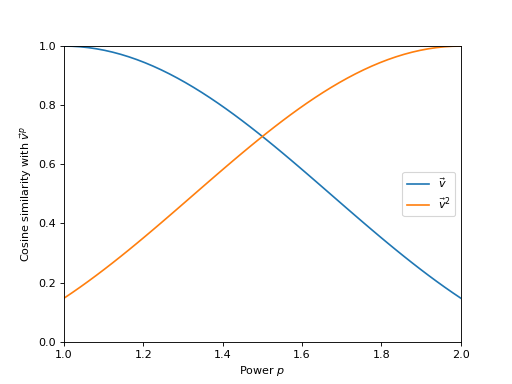The binding of Semantic Pointers is similar to multiplication. When you multiply a number repeatedly with itself, you get a power of this number, e.g. $$x \cdot x \cdot x = x^3$$. This concept translates to binding:

$\vec{v} \circledast \vec{v} \circledast \vec{v} = \vec{v}^3$

When working with SemanticPointer instances, you can just use Python’s power operator **; algebras provide a binding_power method:

assert np.allclose((pointer * pointer * pointer).v, (pointer ** 3).v)
assert np.allclose((pointer * pointer * pointer).v, algebra.binding_power(pointer.v, 3))


The exponent of such a power may also be a real (as in non-integer) number under certain conditions, e.g. $$\vec{v}^{1.23}$$. We call this fractional binding (or fractional binding powers) as the vector will be bound only “partially” to itself. As you let the exponent $$p$$ change from 1 to 2, the binding power $$\vec{v}^p$$ will transition continuously from $$\vec{v}$$ to $$\vec{v}^2$$.Fractional binding is useful because it allows encoding real-valued numbers. One application, where this is required, is the representation of continuous space [komer2019].

## Semantic Pointer signs¶

Fractional exponents can only be used with certain Semantic Pointers. Real numbers can serve here as an analogy again. Consider, for example, $$(-1)^{0.5} = \sqrt{-1}$$: there is no solution within the real numbers itself (you’d need to expand the domain to complex numbers). Fractional exponents require a non-negative sign of the base. The concept of a sign can be translated to Semantic Pointers, albeit a bit more complex, and a fractional binding requires a Semantic Pointer with non-negative sign.

A sign of a Semantic Pointer can not just be positive, negative, or zero, but also indefinite. There might also be multiple types of negative signs. Thus, the binding of two Semantic Pointers with negative signs might not be positive. Only the binding of two Semantic Pointers with the same type of sign will yield a positive Semantic Pointer.

Like the sign of a number has a corresponding number (+1 for the positive sign, —1 for the negative sign, 0 for zero), certain Semantic Pointers will correspond to the Semantic Pointer signs. Binding a Semantic Pointer with its sign vector will give a positive Semantic Pointer.

How exactly the signs work depends on the algebra. See the documentation of HrrSign, VtbSign, and TvtbSign for more details.

To determine the sign of a Semantic Pointer use the sign method:

sign = pointer.sign()
print("Positive?", sign.is_positive())
print("Negative?", sign.is_negative())

Positive? False
Negative? True


A sign will also correspond to a specific Semantic Pointer. Binding a Semantic Pointer to the inverse sign Semantic Pointer will give a positive version of the bound Semantic Pointer in most algebras.

abs_pointer = ~pointer.sign().to_semantic_pointer() * pointer
print("Positive?", abs_pointer.sign().is_positive())

Positive? True


Hint

This is analogous to the sign of complex numbers:

$\mathrm{sign}(z) = \frac{z}{\mathrm{abs}(z)}$

By cross-multiplying we get:

$\mathrm{abs}(z) = \mathrm{sign}^{-1}(z) \cdot z$

One can also use the abs method to obtain a positive Semantic Pointer based on a given Semantic Pointer. If a new positive Semantic Pointer, without relation to an existing Semantic Pointer, is needed, the VectorsWithProperties generator can be used:

from nengo_spa.algebras import CommonProperties
from nengo_spa.vector_generation import VectorsWithProperties

gen = VectorsWithProperties(d, {CommonProperties.POSITIVE}, algebra=algebra)
positive_pointer = spa.SemanticPointer(next(gen), algebra=algebra)

print("Positive?", positive_pointer.sign().is_positive())

Positive? True


## Desirable properties for exponentiated Semantic Pointers¶

When increasing the exponent in a power of a number, the result will approach either 0 or grow without bound ($$\lim_{p \rightarrow \infty} x^p = 0$$ if $$0 \leq x < 1$$, $$\lim_{p \rightarrow \infty} x^p = \infty$$ if $$x > 1$$). The same can happen for the vector length of the binding power of a Semantic Pointer. This might be undesirable (e.g. for representation in neurons). Using a unitary Semantic Pointer ensures that the vectors length will stay constant. The VectorsWithProperties generator can be used to create positive unitary Semantic Pointers:

from nengo_spa.algebras import CommonProperties
from nengo_spa.vector_generation import VectorsWithProperties

gen = VectorsWithProperties(
d,
{CommonProperties.POSITIVE, CommonProperties.UNITARY},
algebra=algebra
)
positive_unitary_pointer = spa.SemanticPointer(next(gen), algebra=algebra)


Note that pointer.abs().unitary() or pointer.unitary().abs() is not guaranteed to work because the operation of making a Semantic Pointer unitary (positive) can destroy the property of being positive (unitary) if not both constraints are taken into account at the same time.

The binding powers of a positive, unitary Semantic Pointer move around a multidimensional circle. A negative Semantic Pointer will jump between such multidimensional circles with each binding (similar to the powers of a negative number that are alternating between positive and negative numbers).

## Exponentiation laws¶

The usual exponentiation laws do not hold in general for binding powers, i.e. $$(\vec{v}^a)^b \ne \vec{v}^{a \cdot b}$$ and $$\vec{v}^a \cdot \vec{v}^b \ne \vec{v}^{a + b}$$. For a specific algebra, exponentiation laws might hold under certain conditions. See HrrAlgebra.binding_power, VtbAlgebra.binding_power, and TvtbAlgebra.binding_power for details.

## Negative exponents and approximate inverses¶

For real numbers, an exponent of —1 is equivalent to the multiplicative inverse. Semantic Pointer binding powers work similar, however, an exponent of —1 represents the approximate inverse here. Thus, the identity $$(\vec{v}^a)^b = \vec{v}^{a \cdot b}$$ only holds for unitary Semantic Pointers $$\vec{v}$$.